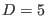# 9 Conclusion

The most important conclusion of this paper is that under the hypotheses of analyticity in the coupling constant, spacetime locality, Lorentz covariance, and Poincaré invariance of the deformations, combined with the preservation of the number of derivatives on each field, the dual formulation of linearized gravity inallows for the first time nontrivial couplings to another theory, namely with a topological BF model, whose field spectrum consists in a scalar field, two sorts of one-forms, two types of two-forms, and a three-form. The deformed Lagrangian contains mixing-component terms of order one in the deformation parameter that couple the massless tensor field with the mixed symmetrymainly to one of the two-forms and to the three-form from the BF sector. There appear some self-interactions in the BF sector at order two in the coupling constant that are strictly due to the presence of the tensor field with the mixed symmetry. One of the striking features of the deformed model is that the gauge transformations of all fields are deformed. This is the first case where the gauge transformations of the tensor field with the mixed symmetrydo change with respect to the free ones (by shifts in some of the BF gauge parameters). All the ingredients of the gauge structure are modified by the deformation procedure: the gauge algebra becomes open and the reducibility relations hold on-shell.

Ashkbiz Danehkar
2018-03-26